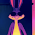## Tuesday, April 9, 2019

1.This comment has been removed by the author.

2.3.4.Jets win superbowl 54

1.5.A = 36 B = 37...

1 + 2 + 3.....+ 36 = 666 as we all know.

6 x 6 x 6 = 2 1 6

The First occurrence of the digits 2 1 6 in Pi are followed
by these digits,

2 1 6 4 2 0 1 9 8 9

2019 is 1989 yrs from The Crucifixion of Jesus Christ

4201 - 1 = 906{Jesus Christ/a=6 b=12...) + 3294{this is a date

a=6 b=12 c=18....
Thursday November Seventh Two Thousand Nineteen = 3294

a=36 b=37 c=38...
Thursday November Seventh Two Thousand Nineteen = 2019
This will be THE Date of 2019 which is 6+6+6 yrs after 911

a=36 b=37 c=38...
Two Thousand Nineteen = 911

6."ISRAEL" = 541 in Greek

Using increments of 6{a=6 b=12...)
"May Fourteenth Two Thousand Eighteen" = 2 4 2 4

At the first occurrence of 2 4 2 4 in Pi these digits fpllow,
2 4 2 4 5 4 1

541 days after May 14th 2018(70th anniversary) is
Nov.7th 2019

7.Enochs Rapture year 987 yrs after Adams creation,
was chosen for a reason!

9+8+7 = 8+8+8
9x8x7 = 504
987 divides evenly by 7

There is only one number greater than 987,with digits
that come this close to having those 3 features,

1+9+9+5 = 8+8+8{Jesus = 888 Gr.
1x9x9x5 = 405
1995 divides evenly by 7

Ivan Panin chronology,

Flood Ends 1657 + 2368(J.C.)yrs + 1995 yrs = NOW

The First Verse of Bible chapter 667,
"Who is as the wise man, and who knoweth
the interpretation of a thing"
= [ 2019 + 1995 ]

When 1995 occurs for the First time in the
digits of Pi, this is what you see,
201995

In Greek,
"The Bread of God" = 1995

8.123 45 6** 789 0

123 + 666 = 789
6x6x6 = 216
666 - 216 = 450{Noahs Ark, God wanted us to know for a reason,
was 450 ft long

The 450 ft Ark is LIFT UP ABOVE THE EARTH after the first
*176 verses* of The Bible are completed.

The instructions to COUNT 6 6 6 begin for the last
*176 verses* of The Bible

9.Genesis Chapter Seven Verse Seventeen( 2352 )
+
Revelation Chapter Thirteen Verse Eighteen( 2598*)

= 1656(yr of Flood) + 3294{Thu.Nov.7th 2019)

"November Seventh Two Thousand Nineteen" = 2598*

A=6 B=12 C=18....Z=156 / G O D = 156